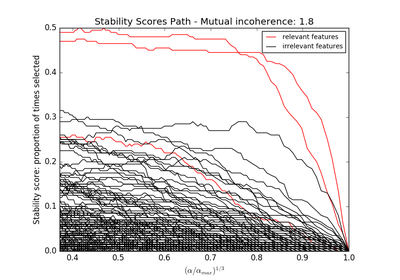# `sklearn.linear_model`.RandomizedLasso¶

class `sklearn.linear_model.``RandomizedLasso`(alpha='aic', scaling=0.5, sample_fraction=0.75, n_resampling=200, selection_threshold=0.25, fit_intercept=True, verbose=False, normalize=True, precompute='auto', max_iter=500, eps=2.2204460492503131e-16, random_state=None, n_jobs=1, pre_dispatch='3*n_jobs', memory=Memory(cachedir=None))[source]

Randomized Lasso.

Randomized Lasso works by subsampling the training data and computing a Lasso estimate where the penalty of a random subset of coefficients has been scaled. By performing this double randomization several times, the method assigns high scores to features that are repeatedly selected across randomizations. This is known as stability selection. In short, features selected more often are considered good features.

Read more in the User Guide.

Parameters: alpha : float, ‘aic’, or ‘bic’, optional The regularization parameter alpha parameter in the Lasso. Warning: this is not the alpha parameter in the stability selection article which is scaling. scaling : float, optional The s parameter used to randomly scale the penalty of different features (See User Guide for details ). Should be between 0 and 1. sample_fraction : float, optional The fraction of samples to be used in each randomized design. Should be between 0 and 1. If 1, all samples are used. n_resampling : int, optional Number of randomized models. selection_threshold: float, optional : The score above which features should be selected. fit_intercept : boolean, optional whether to calculate the intercept for this model. If set to false, no intercept will be used in calculations (e.g. data is expected to be already centered). verbose : boolean or integer, optional Sets the verbosity amount normalize : boolean, optional, default False If True, the regressors X will be normalized before regression. This parameter is ignored when fit_intercept is set to False. When the regressors are normalized, note that this makes the hyperparameters learned more robust and almost independent of the number of samples. The same property is not valid for standardized data. However, if you wish to standardize, please use preprocessing.StandardScaler before calling fit on an estimator with normalize=False. precompute : True | False | ‘auto’ Whether to use a precomputed Gram matrix to speed up calculations. If set to ‘auto’ let us decide. The Gram matrix can also be passed as argument. max_iter : integer, optional Maximum number of iterations to perform in the Lars algorithm. eps : float, optional The machine-precision regularization in the computation of the Cholesky diagonal factors. Increase this for very ill-conditioned systems. Unlike the ‘tol’ parameter in some iterative optimization-based algorithms, this parameter does not control the tolerance of the optimization. n_jobs : integer, optional Number of CPUs to use during the resampling. If ‘-1’, use all the CPUs random_state : int, RandomState instance or None, optional (default=None) If int, random_state is the seed used by the random number generator; If RandomState instance, random_state is the random number generator; If None, the random number generator is the RandomState instance used by np.random. pre_dispatch : int, or string, optional Controls the number of jobs that get dispatched during parallel execution. Reducing this number can be useful to avoid an explosion of memory consumption when more jobs get dispatched than CPUs can process. This parameter can be: None, in which case all the jobs are immediately created and spawned. Use this for lightweight and fast-running jobs, to avoid delays due to on-demand spawning of the jobs An int, giving the exact number of total jobs that are spawned A string, giving an expression as a function of n_jobs, as in ‘2*n_jobs’ memory : Instance of joblib.Memory or string Used for internal caching. By default, no caching is done. If a string is given, it is the path to the caching directory. scores_ : array, shape = [n_features] Feature scores between 0 and 1. all_scores_ : array, shape = [n_features, n_reg_parameter] Feature scores between 0 and 1 for all values of the regularization parameter. The reference article suggests `scores_` is the max of `all_scores_`.

Notes

See examples/linear_model/plot_sparse_recovery.py for an example.

References

Stability selection Nicolai Meinshausen, Peter Buhlmann Journal of the Royal Statistical Society: Series B Volume 72, Issue 4, pages 417-473, September 2010 DOI: 10.1111/j.1467-9868.2010.00740.x

Examples

```>>> from sklearn.linear_model import RandomizedLasso
>>> randomized_lasso = RandomizedLasso()
```

Methods

 `fit`(X, y) Fit the model using X, y as training data. `fit_transform`(X[, y]) Fit to data, then transform it. `get_params`([deep]) Get parameters for this estimator. `get_support`([indices]) Return a mask, or list, of the features/indices selected. `inverse_transform`(X) Transform a new matrix using the selected features `set_params`(\*\*params) Set the parameters of this estimator. `transform`(X) Transform a new matrix using the selected features
`__init__`(alpha='aic', scaling=0.5, sample_fraction=0.75, n_resampling=200, selection_threshold=0.25, fit_intercept=True, verbose=False, normalize=True, precompute='auto', max_iter=500, eps=2.2204460492503131e-16, random_state=None, n_jobs=1, pre_dispatch='3*n_jobs', memory=Memory(cachedir=None))[source]
`fit`(X, y)[source]

Fit the model using X, y as training data.

Parameters: X : array-like, shape = [n_samples, n_features] Training data. y : array-like, shape = [n_samples] Target values. self : object Returns an instance of self.
`fit_transform`(X, y=None, **fit_params)[source]

Fit to data, then transform it.

Fits transformer to X and y with optional parameters fit_params and returns a transformed version of X.

Parameters: X : numpy array of shape [n_samples, n_features] Training set. y : numpy array of shape [n_samples] Target values. X_new : numpy array of shape [n_samples, n_features_new] Transformed array.
`get_params`(deep=True)[source]

Get parameters for this estimator.

Parameters: deep : boolean, optional If True, will return the parameters for this estimator and contained subobjects that are estimators. params : mapping of string to any Parameter names mapped to their values.
`get_support`(indices=False)[source]

Return a mask, or list, of the features/indices selected.

`inverse_transform`(X)[source]

Transform a new matrix using the selected features

`set_params`(**params)[source]

Set the parameters of this estimator.

The method works on simple estimators as well as on nested objects (such as pipelines). The latter have parameters of the form `<component>__<parameter>` so that it’s possible to update each component of a nested object.

Returns: self :
`transform`(X)[source]

Transform a new matrix using the selected features

## Examples using `sklearn.linear_model.RandomizedLasso`¶Sparse recovery: feature selection for sparse linear models